Fenrier Lab

# Java HashMap源码分析(1)

### 哈希表索引下标计算

public class hashmap<K, V>{

private node<K, V>[] table;

public V get(K key) {
int index = hash(key);
return table[index].value;
}

public void put(K key, V value) {
int index = hash(key);
table[index] = new node(key, value);
}
static class node<K, V> {
K key;
V value;
node(K key, V value) {
this.key = key;
this.value = value;
}
}
}


hashmap用内部类 node 来管理键值对，然后使用 node 数组 table 作为键值对容器，之所以用“节点”这个词，是因为在必要时要将这个块扩展为链表或者树，这将在后面讨论。这里的hash函数，接受key，然后返回对应的索引，并且不同的key对应不同的索引，否则数据就被后来者覆盖了，一种很自然的想法是利用Java对象自带的哈希码方法，即hashCode()，来实现这一功能。但问题在于hashCode()计算的值实在太大，总是开辟这样大的数组空间显得不现实。于是HashMap的设计者就想到一个简单粗暴的方法，使用不那么大的数组容量n，然后将哈希码与n - 1作&运算。

h = key.hashCode();
index = h & (n - 1);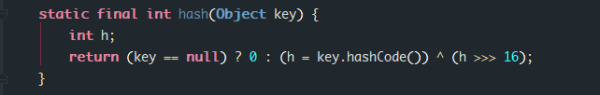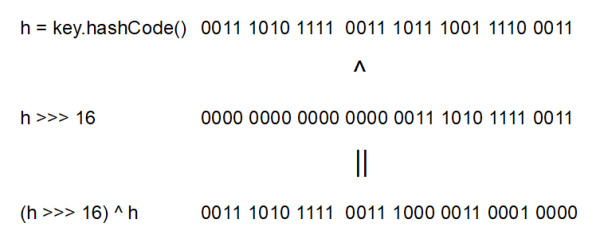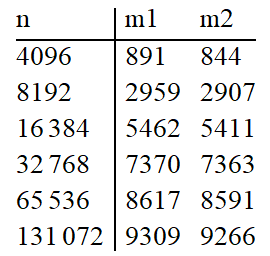There is a tradeoff between speed, utility, and quality of bit-spreading. Because many common sets of hashes are already reasonably distributed (so don’t benefit from spreading), and because we use trees to handle large sets of collisions in bins, we just XOR some shifted bits in the cheapest possible way to reduce systematic lossage, as well as to incorporate impact of the highest bits that would otherwise never be used in index calculations because of table bounds.

static class node<K, V> {
final int hash;
final K key;
V value;
node(int hash, K key, V value) {
this.hash = hash;
this.key = key;
this.value = value;
}
}


### 存入数据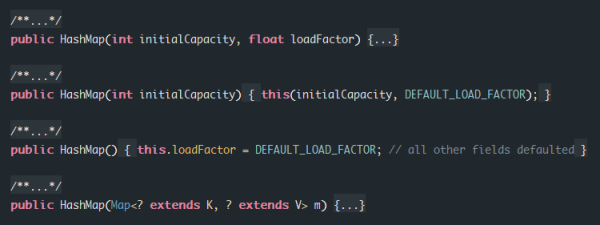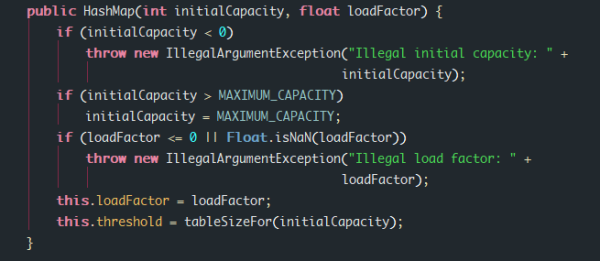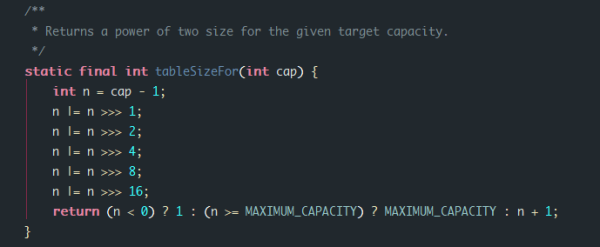public hashmap(int initialCapacity, float loadFactor) {
if(initialCapacity < 0) {
throw new IllegalArgumentException("Illegal initial capacity: " + initialCapacity);
}
}
table = new node[tableSizeFor(initialCapacity)];
}

}

public hashmap() {
}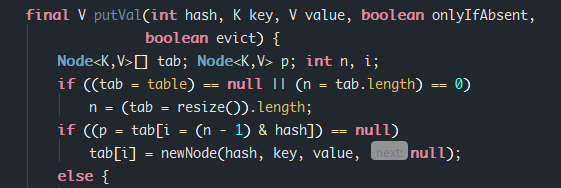putVal 方法接受前面计算的 hash 值，以及一组键值对，首先判断容器数组是否为空，如果是，则先调用 resize 方法初始化容器数组。如果不为空，则判断对应索引上是否有值，如果没有，则将数据放到该位置，如果有，则使用链表或者二叉树来存储相同索引数据，现在先不管这种情况。这里值得注意的是 resize 方法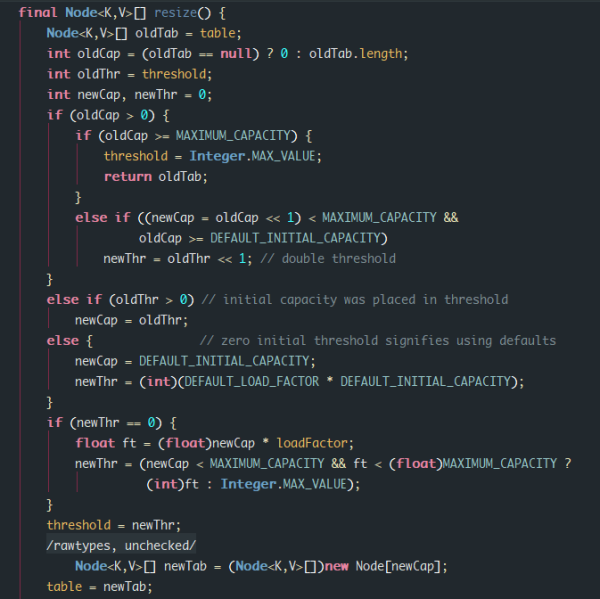1. 方法内首先检查旧容器数组的大小是否为0，如果大于0，则新的数组容量翻倍，并且如果旧数组的容量大于等于默认值，则新的threshold 也翻倍。这种情况对应于HashMap对象已经使用了put方法的情况，因为一开始数组容量一定是等于0的（当然这是在只考虑前三个构造方法的假定下）。
2. 如果旧数组容量等于0，但是 threshold 大于0，那么就将threshold的值作为新的数组容量，这种情况对应于调用 HashMap(int initialCapacity, int loadFactor) 或者 HashMap(int initialCapacity) 新建对象，但是还没有进行 put 操作的情况，这时的 threshold 一定是2的幂，所以可以直接作为数组容量。
3. 如果数组容量和 threshold 都等于0，则用默认值作为数组容量，threshold 也用默认负载因子初始化。这种情况对应于调用 HashMap() 新建对象，但是还没进行 put 操作的情况。

hash = 0000abcd

indexOld = hash & (n1 - 1) = 0000abcd & 00001111 = 0000a’b’c’d’

indexNew = hash & (n - 1) = 0000abcd & 00011111 = 0000a’b’c’d’

indexOld = hash & (n1 - 1) = 0001abcd & 00001111 = 0000a’b’c’d’

indexNew = hash & (n - 1) = 0001abcd & 00011111 = 0001a’b’c’d’

public void resize() {
int capacity = table.length;
int newCap;
if(capacity > 0) {
newCap = capacity << 1;
}
else {
newCap = DEFAULT_INITIAL_CAPACITY;
}

node<K, V>[] newTab = (node<K, V>[])new node[newCap];

for(int i = 0; i < capacity; i++) {
node<K, V> e = table[i];
if(e != null) {
//判断hash的标志位是否为0
if((e.hash & capacity) == 0) {
newTab[i] = e;
}else{
newTab[i + capacity] = e;
}
}
}

table = newTab;
}


public hashmap put(K key, V value) {
if(table.length == 0 || size >= (int) (table.length * loadFactor)) {
resize();
}
int hash = hash(key);
table[hash & (table.length - 1)] = new node<>(hash, key, value);
size++;
return this;
}


### 查询数据

public V get(K key) {
int index = hash(key) & (table.length - 1);
return table[index].value;
}


### 遇到相同 hash 情况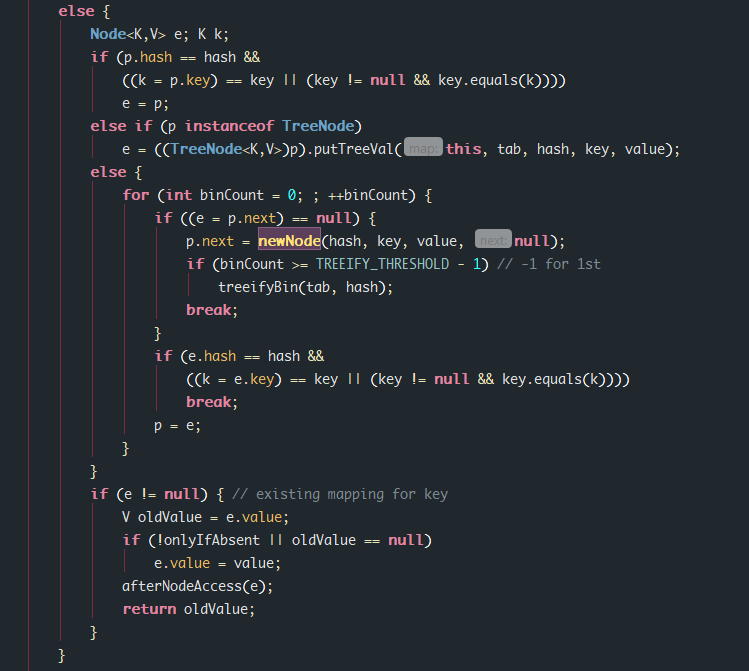1. 如果新插入数据的 hash 值等于已经存在的数据 p 的 hash 值，并且两者的 key 相同，这种情况其实是在更新键值，可以直接覆盖旧数据。
2. 如果已有数据 p 是一个树节点，那么新数据就需要插入到树里面。
3. 最后一种情况意味着 p 是一个链表节点，如果发现新数据的 key 其实就是链表中某个节点的 key，则用新的value覆盖，如果一直没发现同 key 数据，则需要将新数据插入到链表末尾。binCount 的终值记录了链表长度，如果发现链表长度超过了阈值 TREEIFY_THERSHOLD ，那么就把链表转换成二叉树（确切的说是一棵红黑树）。

static class node<K, V>{

//code

node<K, V> next;

//code
}



public hashmap put(K key, V value) {
if(table.length == 0 || size >= (int) (table.length * loadFactor)) {
resize();
}
int hash = hash(key);
int index = hash & (table.length - 1);
node<K, V> p = table[index];
K k;
if(p != null) {
//如果新旧数据的键相同，则只需更新 value
if(p.hash == hash && ((k = p.key) == key || (key != null && key.equals(k)))) {
p.value = value;
}else {
node<K, V> e;
//遍历节点p开头的链表，如果在链表中发现key，则对value进行更新。否则将新的键值对插入链表末尾
while(true){
e = p.next;
if(e == null) {
p.next = new node<>(hash, key, value);
size++;
break;
}
if(e.hash == hash && ((k = e.key) == key || key != null && key.equals(k))) {
e.value = value;
break;
}
p = e;
}
}
}else{
table[index] = new node<>(hash, key, value);
size++;
}
if(size >= table.length * loadFactor) {
resize();
}
return this;
}